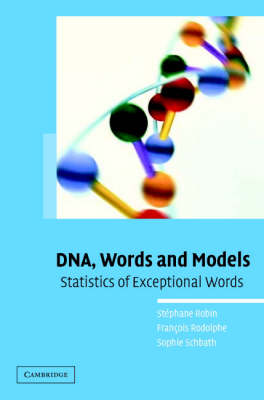•# DNA, Words and Models: Statistics of Exceptional Words (Hardback)

(author), (author), (author)
£59.99
Hardback 158 Pages / Published: 13/10/2005
• We can order this

Usually dispatched within 3 weeks

An important problem in computational biology is identifying short DNA sequences (mathematically, 'words') associated to a biological function. One approach consists in determining whether a particular word is simply random or is of statistical significance, for example, because of its frequency or location. This book introduces the mathematical and statistical ideas used in solving this so-called exceptional word problem. It begins with a detailed description of the principal models used in sequence analysis: Markovian models are central here and capture compositional information on the sequence being analysed. There follows an introduction to several statistical methods that are used for finding exceptional words with respect to the model used. The second half of the book is illustrated with numerous examples provided from the analysis of bacterial genomes, making this a practical guide for users facing a real situation and needing to make an adequate procedure choice.

Publisher: Cambridge University Press
ISBN: 9780521847292
Number of pages: 158
Weight: 391 g
Dimensions: 235 x 156 x 16 mm

MEDIA REVIEWS
'For statisticians with a little background in biology, this book delivers a very readable presentation on the analysis of DNA sequences to determine whether a motif is of statistical significance due to its overabundance (or under-abundance) in terms of frequencies or location. This book is concise but sufficiently detailed. Biologists without a background in mathematical statistics may find the learning curve a little steep but tractable. The authors' continuous use of practical examples will be greatly appreciate by biologists and statisticians alike. This book is one of a kind, and I recommend it to any statistician interested in learning about DNA sequences and motifs.' Journal of the American Statistical Association
"For statisticians with a little background in biology, this book delivers a very readable presentation on the analysis of DNA sequences to determine whether a motif is of statistical significance due to its overabundance (or underabundance) in terms of frequencies or location. This book is concise but sufficiently detailed. Biologists without a background in mathematical statistics may find the learning curve a little steep but tractable. The authors' continuous use of practical examples will be greatly appreciated by biologists and statisticians interested in learning about DNA sequences and motifs." J. Wade Davis, University of Missouri-Columbia, Journal of the American Statistician
"... A welcome introduction to word analysis..." Daniel M. Burns, Jr., Mathematical Reviews